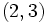# Characteristicity is not finite-relative-intersection-closed

This article gives the statement, and possibly proof, of a subgroup property (i.e., characteristic subgroup) not satisfying a subgroup metaproperty (i.e., finite-relative-intersection-closed subgroup property).
View all subgroup metaproperty dissatisfactions | View all subgroup metaproperty satisfactions|Get help on looking up metaproperty (dis)satisfactions for subgroup properties
Get more facts about characteristic subgroup|Get more facts about finite-relative-intersection-closed subgroup property|

## Statement

### Verbal statement

It is possible to have a group$G$ with subgroups$H,K,L$, such that$H \le L, K \le L$,$H$ characteristic in$G$ and$K$ characteristic in$L$, but$H \cap K$ not characteristic in$G$.

## Example

### Example where$L = HK$

Further information: symmetric group:S4, dihedral group:D8, subgroup structure of symmetric group:S4

Suppose$G$ is the symmetric group on the set$\{ 1,2,3,4 \}$. Define:$H := \{ (), (1,2)(3,4), (1,3)(2,4), (1,4)(2,3) \}, K := \{ (), (1,2,3,4), (1,3)(2,4), (1,4,3,2) \}$

and define$L = HK$, explicitly,$L$ is a$2$-Sylow subgroup of$G$ isomorphic to the dihedral group:$L := \{ (), (1,2,3,4), (1,3)(2,4), (1,4,3,2), (1,2)(3,4), (1,4)(2,3), (1,3), (2,4) \}$.

Then:

•$H$ is characteristic in$G$: In fact, it is the only normal subgroup of order$4$ in$G$, and also equals the second commutator subgroup of$G$.
•$K$ is characteristic in$L$: In fact,$K$ is an isomorph-free subgroup of$L$.
•$H \cap K$ is not characteristic in$G$: The intersection$H \cap K$ is the two-element subgroup$\{ (), (1,3)(2,4)\}$, which is not characteristic -- in fact, it is not even normal in$G$, as it is not invariant under conjugation by$(2,3)$.

### Example where$K \le H$

Further information: symmetric group:S4, dihedral group:D8, subgroup structure of symmetric group:S4

Suppose$G$ is the symmetric group on the set$\{ 1,2,3,4 \}$. Define:$H := \{ (), (1,2)(3,4), (1,3)(2,4), (1,4)(2,3) \}, K := \{ (), (1,3)(2,4) \}$

and$L$ is a$2$-Sylow subgroup of$G$ isomorphic to the dihedral group, containing$H$:$L := \{ (), (1,2,3,4), (1,3)(2,4), (1,4,3,2), (1,2)(3,4), (1,4)(2,3), (1,3), (2,4) \}$.

Then:

•$H$ is characteristic in$G$: In fact, it is the only normal subgroup of order$4$ in$G$, and also equals the second commutator subgroup of$G$.
•$K$ is characteristic in$L$: In fact,$K$ is the center of$L$.
•$H \cap K$ is not characteristic in$G$: The intersection$H \cap K$ is the two-element subgroup$K = \{ (), (1,3)(2,4)\}$, which is not characteristic -- in fact, it is not even normal in$G$, as it is not invariant under conjugation by$(2,3)$.